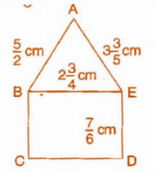# 5. Find the perimeters of (ii) the rectangle BCDE in this figure. Whose perimeter is greater?The perimeter of  = AB + BE + AE

Noe, The LCM of 2,4 and 5 is 20. So let's make the denominator of all fraction, 20.

So,

The perimeter of  :

Now,

The perimeter of rectangle BCDE = 2 x ( BE + ED )

The LCM of 4 and 6 is 12. So let's make the denominator of both fractions equal to 12.

Hence The perimeter of the Triangle is  and the perimeter of Rectangle is  .

Now, we have

LCM of 20 and 6 is 60, so let's make denominator of both fraction equal to 60.

So,

And

Now, Since 531 > 470

Area of Triangle > Area of Rectangle.

## Related Chapters

### Preparation Products

##### JEE Main Rank Booster 2021

This course will help student to be better prepared and study in the right direction for JEE Main..

₹ 13999/- ₹ 9999/-
##### Rank Booster NEET 2021

This course will help student to be better prepared and study in the right direction for NEET..

₹ 13999/- ₹ 9999/-
##### Knockout JEE Main April 2021 (Easy Installments)

An exhaustive E-learning program for the complete preparation of JEE Main..

₹ 4999/-
##### Knockout NEET May 2021

An exhaustive E-learning program for the complete preparation of NEET..

₹ 22999/- ₹ 14999/-